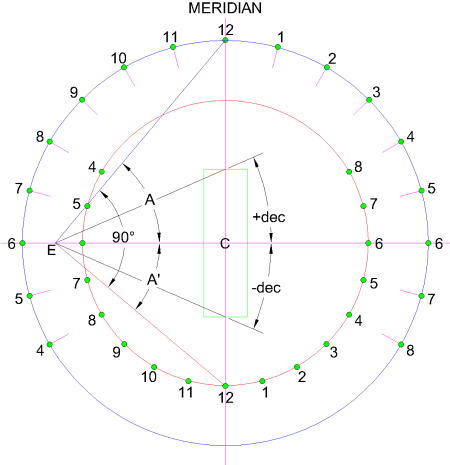The Sundial Primer created by Carl SabanskiThe Sundial Primer Index
 Double Foster-Lambert Sundial Foster-Lambert Sundial: a form of equatorial projection dial, with the projection arranged to produce a circular ring of equiangular hour points. The Foster-Lambert sundial can be designed to be self-aligning by incorporating two sundials on the same dial plate using a common scale of dates. Once constructed and positioned correctly, the dial plate is rotated until both sundials indicate the same time.This design incorporates two gnomons as illustrated in Figure 2.  One gnomon, FL1, lies half way between a polar pointing gnomon "P" and a vertical gnomon "Z". as was discussed previously. Its position is determined by the calculation of the angle "A" relative to the horizontal dial plate.A = (90 + ø) / 2 where ø is the latitude. The span of the gnomon's angle "A" is from 45º at a latitude of 0º to 90º at a latitude of 90º. The second gnomon, FL2, is rotated 90º from FL1. Its position is determined by the calculation of the angle "A'" relative to the horizontal dial plate. A' = (90 - ø) / 2 The span of angle "A" for gnomon FL1 is from 45º at a latitude of 0º to 90º at a latitude of 90º. The span of angle "A'" for gnomon FL2 is from 45º at a latitude of 0º to 0º at a latitude of 90º. The two gnomons lie on the meridian line.Figure 1: Double Foster-Lambert Sundial Gnomons (CAD) Figure 2 illustrates the design layout for the sundial. The outer sundial is designed as discussed on the Foster-Lambert Sundial page. This sundial is designed using the blue line representing gnomon FL1. Rotate this line 90º clockwise to locate the position of the second gnomon FL2, which is shown as the red line. The point where this line crosses the meridian line will establish the radius of the circle for the inner sundial. The hour points can be placed on the circle for the inner sundial as they were for the outer one. Note the differences in the positioning and numbering of the hour points. The design of the outer sundial will also establish the position of "E". The position of "E" from the centre "C" is calculated as follows:E = R / tan A = R * tan (90 - A) where "R" is the radius of the outer circle.Figure 2: Double Foster-Lambert Sundial Layout (CAD) The distance from the sundial's centre "C" to the intersection point "Y" on the meridian line for any desired date is calculated as follows:Y = E * tan (dec)where "dec" is the sun's declination.Figure 2 shows the scale points for the two solstices. As stated earlier the two sundials use a common scale of dates and as a result a common point "E". This is evident from the design in Figure 2.The radius "R'" of the inner circle can be calculated as follows:R' = E * tan A'The radius of the inner circle will equal the outer circle at a latitude of 0º and will be 0 at a latitude of 90º. The diameter of the inner circle will equal the size of the date scale at a latitude calculated as follows:R' =  Y (dec = 23.44)E * tan A' = E * tan (dec)A' = dec(90 - ø) / 2 = 23.44 ø = 90 - (23.44 * 2) = 43.12º At latitudes lower than 43.12º the inner circle will be outside the scale of dates and at higher latitudes it will be inside. When the inner circle becomes smaller than the scale of dates it may become difficult to draw the inner sundial clearly. An option is to design a sundial for a lower latitude, which will result in a larger inner circle. The sundial is then tilted by an angle equal to the difference between the actual latitude and the design latitude.Because the hour points are equiangular it is quite simple to correct for the Equation of Time, longitude and daylight saving time. The dial needs to be designed so the hour points of the outer sundial can rotate about the centre "C" independently of the remainder of the dial plate. The outer hour points are then rotated to the required correction. The inner sundial will indicate local apparent or solar time unless the latitude is 0º and the radius of both sundials is the same.Valentin Hristov tells of a way to use this sundial to determine the times of sunrise and sunset on any given day. Place a straight edge on the centre line of the scale of dates at the desired date. Rotate the straight edge until it crosses the hour points, in the morning on the inner and outer sundial, showing the same time. This time will be sunrise. Use the same procedure in the afternoon to find sunset. Don't forget to add the corrections for longitude and the Equation of Time.